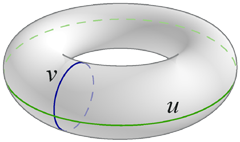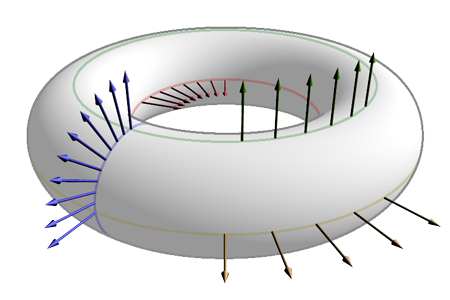]> The Shape Operator of the Torus

# The Shape Operator of the Torus

What's normal here may not be normal there

The shape operator describes how the normal to a surface changes as we move around on the surface. Pretty much everything you could want to know about a surface's curvature is locked up in the shape operator. But, as usual, the torus's shape operator is neglected in the literature.

To find it, we begin with the parameterized surface x:$x u v = x = c + a cos v cos u y = c + a cos v sin u z = a sin v$

The normal to this surface is $N = cos u cos v , sin u cos v , sin v$. Taking the partial derivatives of N with respect to u and v gives the shape operator in those directions:

$− S x u = N u = − sin u cos v , cos u cos v , 0 − S x v = N v = − cos u sin v , − sin u sin v , cos v$

Comparing these to the partial derivatives of the parameterization,

$x u = − c + a cos v sin u , c + a cos v cos u , 0 x v = − a cos u sin v , − a sin u sin v , a cos v$

we find the former are multiples of the latter:

$S x u = − cos v c + a cos v x u S x v = − 1 a x v$

The shape operator is linear, so we can find it not only for the xu and xv directions, but for any direction θ (measured from xv):

$S x = − cos v c + a cos v x u cos θ − 1 a x v sin θ$

From the shape operator, we obtain the Gaussian curvature K (the determinant of the shape operator) and the mean curvature H (the trace of the shape operator).

$K = − cos v c + a cos v 0 0 − 1 a = cos v a c + a cos v H = − c + 2 a cos v 2 a c + a cos v$

## The Geometric Significance of the Shape Operator

For a circle with a normal pointing outward, the numeric coefficient of the shape operator is $− 1 r$, where r is the circle's radius. We can use this to check our solution. Consider the how the normals change in the following illustration.The blue normals lie on a meridian (u constant, v changing), hence they change at a constant rate of $− 1 a$. This is precisely the negative reciprocal of the minor radius of the torus, so that's correct.

The green normals are on the uppermost circle of the torus, where $v = π 2$. The numeric coefficient of the shape operator in the u direction is a multiple of cos v = 0, so the shape operator on this circle in the u direction is zero. As the illustration demonstrates, these normals don't change direction as we move around the circle; they all point straight up (discounting the illustration's perspective, of course).

This makes sense for another reason. The curvature of the torus on its uppermost and lowermost circles is zero. Hence locally along these circles, the torus looks like a cylinder. Since the shape operator of a cylinder in a direction parallel to its axis is zero, we'd expect the shape operator along these circles to be zero as well.

The brown normals lie on the circle v = 0. Working out the shape operator in the xu direction, we find its numeric coefficient to be $− 1 c + a$, the negative reciprocal of the outer equator's radius, as expected.

The last set of normals to check are the red ones on the inner equator. Here v = π, so instead of being negative, the shape operator's numeric coefficient is positive. We can see the difference: instead of diverging like the normals on a meridian or the outer equator, the tips of these normals are closer than their bases. This is a sign that we're on the inside of a circle, where the numeric coefficient of the shape operator is positive. And, being $1 c - a$, it is.

Four checks, four sensible results. Looks like a winner.

If you enjoyed this, you might try verifying that the shape operator takes the form $− 1 p v$ (for some constant p) along the torus's Villarceau circles. That's a level of masochism I'm not prepared to descend to.

Last updated 4 October 2004
http://www.rdrop.com/~half/math/torus/shape.operator.xhtml
All contents released into the public domain by Mark L. Irons